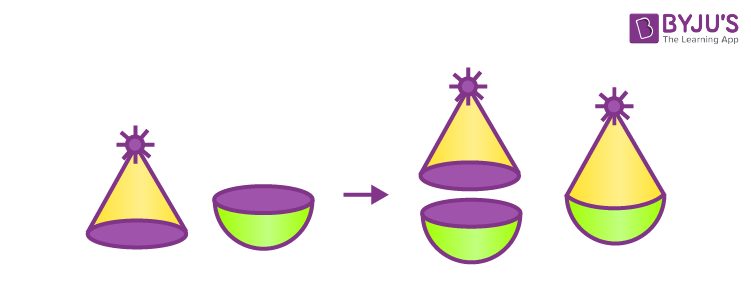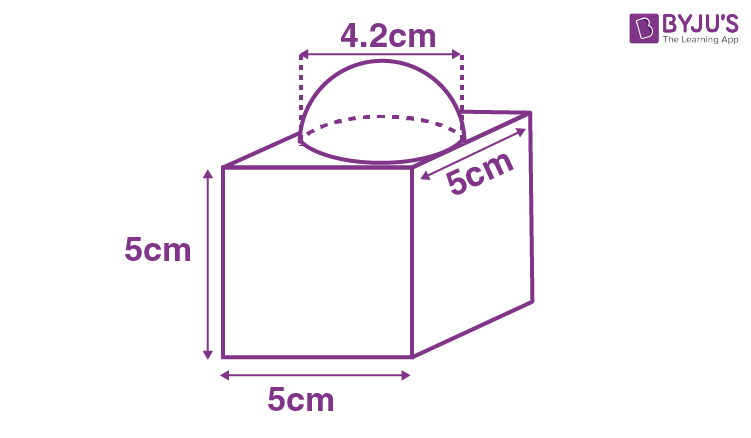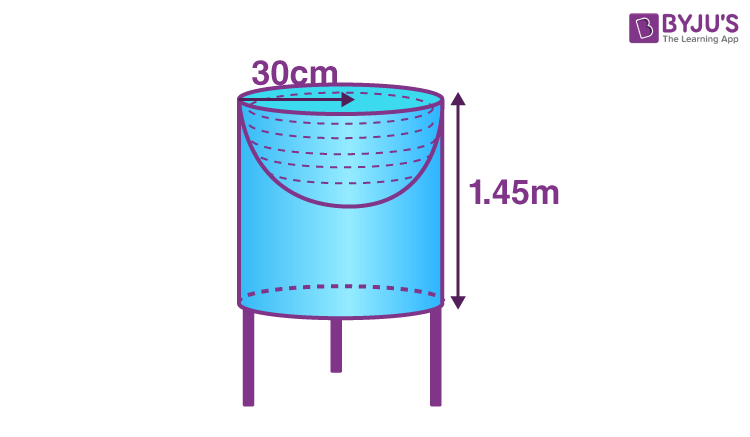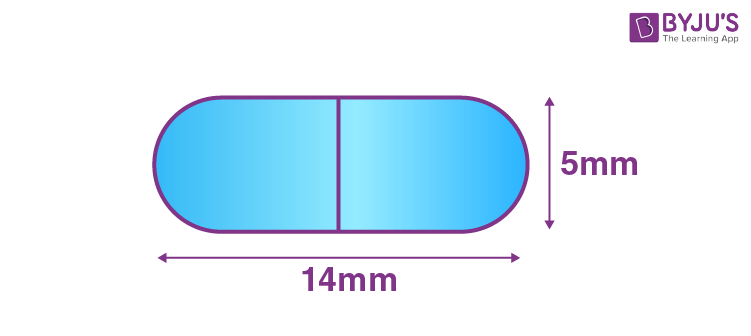# Surface Area of a Combination of Solids

In our daily life activities, we have come across different types of solids that are made up of a combination of two or more basic solids. We are already familiar with surface areas of different solid or three-dimensional shapes such as cube, cuboid, cylinder, cone, sphere, hemisphere and so on. In this article, we will discuss how to find the surface area of the combination of solids with many solved examples in detail.

## Surface Area of a Combination of Solids Formula

The formula to find the surface area of a combination of solids is given as:

TSA of new Solid = Sum of the CSA of each of the individual parts

Where,

TSA = Total Surface Area

CSA = Curved Surface Area.

Now, let us understand how to find the surface area of a combination of solids with the help of an example.

For instance, if we are making a toy by combining the cone and hemisphere, we have to bring the flat faces of the cone and hemisphere together as shown in the figure. In order to have a smooth toy surface, we should take the base radius of the cone equal to the radius of the hemisphere as shown in the figure.Therefore, the surface area of the toy is given by the formula,

The total surface area of the toy = Curved surface area of cone + Curved surface area of hemisphere.

## Surface Area of a Combination of Solids Examples

Example 1:

A block is made up of two solids such as cube and hemisphere as shown in the figure. The base of the block is a cube with an edge 5 cm and the hemisphere fixed on the top has a diameter of 4.2 cm. Find the total surface area of a block (Take π = 22/7).Solution:

Given: Edge of a cube, a = 5 cm.

The total surface area of a cube = 6a2 square units.

TSA of cube = 6(5)2 = 6(25) = 150 cm2.

From the given figure, it is observed that a hemisphere is attached to the part of a cube. Hence, the part of the cube is not included in the surface area.

Therefore, the formula to calculate the surface area of the block is given as:

Surface Area of a block = TSA of cube – Base area of Hemisphere + CSA of hemisphere.

Surface area of a block = 150 – πr2 + 2πr2 cm2

= 150 + πr2 cm2

= 150 + [(22/7)(2.1)(2.1)] cm2 [Since, the diameter of a hemisphere is 4.2 cm)

= 150 + 13.86 cm2

= 163.86 cm2

Therefore, the surface area of a block is 163.86 cm2.

Example 2:

Arjun made a bird-bath for his garden in the cylindrical shape with a hemispherical depression at one end as shown in the figure. The height of the cylinder is 1.45m and its radius is 30 cm. Find the total surface area of the bird-bath and use π = 22/7.Solution:

Let “h” be the height of the cylinder and “r” be the common radius of cylinder and hemisphere.

Therefore, the total surface area of a bird bath = CSA of cylinder + CSA of hemisphere.

TSA of bird-bath = 2πrh + 2πr2 cm2

TSA of bird-bath = 2πr (h+r) cm2

Now, substitute π = 22/7, h = 145 cm and r = 30 cm.

TSA of bird-bath = [2(22/7)(30)] [145+30] cm2

= [2(22/7)(30)] (175)cm2

= 33000 cm2 = 3.3m2

Therefore, the total surface area of a bird-bath is 3.3m2.

### Surface Area of a Combination of Solids Practice Problems

Solve the following problems on the surface area of a combination of solids:

1. A toy is in the shape of a cone of radius 3.5 cm mounted on a hemisphere of the same radius and the total height of the toy is 15.5 cm. Determine the total surface area of a toy.
2. A medicine capsule is in the form of a cylinder with two hemispheres stuck to each other of its ends as shown in the figure. The diameter of the capsule is 5mm and the length of the entire capsule is 14mm. Find the surface area of a medicine capsule.
3.4. A tent is in the cylindrical form surmounted by a conical top. The height and diameter of the cylindrical part are 2.1 m and 4 m respectively, and the slant height of the top is 2.8m. Find the area of the canvas used for making the tent. Also, compute the cost of the canvas of the tent at the rate of Rs. 500 per m2. (Note: The base of the tent should not be covered with canvas.)

Stay tuned with BYJU’S – The Learning App and also learn more Maths-related concepts easily by exploring interesting videos.